Disclaimer: This is an example of a student written essay.

Any scientific information contained within this essay should not be treated as fact, this content is to be used for educational purposes only and may contain factual inaccuracies or be out of date.

# Factors That Affect Resistance and Electric Power

 ✅ Paper Type: Free Essay ✅ Subject: Physics ✅ Wordcount: 1966 words ✅ Published: 9th Mar 2018

A report into the electricity practical investigation and problems

• Samerah Mansha

Abstract:

This experiment was regarding electricity and its features. The experiment determines factors that affect resistance and electric power. The results showed that by increasing the number of bulbs within the electric circuits affected both electric power and resistance value in both series and parallel circuits.

Introduction:

The scope of the investigation was to find out the factors that affect resistance within a circuit by constructing series and parallel circuit’s whilst varying the amount of bulbs used. Additionally, a voltmeter was connected within to measure the electrical potential difference in volts between two points within the circuit. An ammeter was also used to measure the current in amps within the circuit.

Direct current is known as the continuous movement of electrons from areas surrounded with negative charge to areas surrounded with positive charges through a conducting material such as metal wire. Whilst the intensity of current can vary the general direction of movement stays the same throughout. A common example of a direct current is a battery.

Parallel circuits and series circuits are two basic types of electric circuits that can be found within electrical devices. They both differ in terms of where the components are placed within circuitIn series circuits there are no branches present thus components are connected one after another in a single pathway, allowing current to follow the circuit from one side to the other side of cell passing through each of the components, one after the other. In a series circuit, if a lamp breaks or even a component is disconnected, the circuit is broken causing all the components within to stop working. The current found in series circuit is found to be the same anywhere within the circuit, whilst the voltage is shared in ratio to resistance.

Figure: 1 an example of series circuit.

In a parallel circuit, there are multiple pathways between circuit’s beginning and end, whilst different components are connected into different branches of the wire.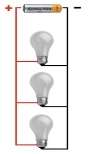Therefore the electric current has more than one route to take, thus circuit has the potential to still function even if one path fails, for example if a lamp breaks or a component is disconnected from one parallel wire, the components on different branches are still able to work therefore parallel circuits are more commonly used in household applications. Regardless of how many different paths the circuit has, the total voltage stays the same, as the voltage across each pathway equals to the supply voltage. The current through parallel circuits splits up as it passes through the passageways.

Figure: 2 – an example of a parallel circuit

Electromotive force abbreviated as e.m.f is when charge flows through a cell or battery, it is given energy.The number of joules of energy given to each coulomb of charge that passes through the cell is known as called e.m.f. of cell and is measured in volts (V). It is equal to the potential difference across the terminals of the cell when no current is flowing. Examples of sources of e.m.f are cells, batteries and generator

If you need assistance with writing your essay, our professional essay writing service is here to help!

Potential difference (p.d) is known as the amount of electrical energy that is changed into other forms of energy per coulomb of charge. In circuits there are components that put energyinto the circuit and components that take energyout. Any device putting energy into circuit is providing an e.m.f whilst any device taking energy out has p.dacross it.The p.d across an electrical component is needed to make current flow in it. Cells or batteries usually are responsible for the p.d needed. P.d is measured in volts using a voltmeter.

Electrical cells are made from materials and chemicals, which have some sort of resistance. Therefore, a batteries and cells must have resistance known asinternal resistance which ismeasured in ohms. When electricity flows round a circuit the internal resistance of the cell itself causes a drop in soruice of voltage whilst resisting the flow of current thusthermalenergy is wasted in the cell itself.

Ohms law is commonly used to portray that current flowing through a metal wire is proportional to the potential difference across it, providing that temperature remains constant.

Resistance is measured in ohms.Ωrthus greater the number of ohms, the greater the resistance.

The relationship between voltage, current and resistance is V= IR, Potential difference (volt, V) = current (ampere, A) × resistance (ohm,Ω).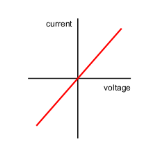Figure: 3 – Graph showing the relationship between current and voltage following Ohm’s Law that electric current is proportional to voltage and inversely proportional to resistance Electric power is the rate of energy consumption and the way electric energy is transferred n within an electrical circuit. The electric power is measured in watts.

Planning:

Equipment:

• Locktronics electricity kit
• Bulbs
• Ammeter
• Voltmeter
• Wires
• Electric Source

Safety Measures:

As the mains voltage electricity was used throughout the experiment, it was dangerous. Thus significant risk of electrocution may occur if mains voltage electricity is allowed to pass through the body. The mains voltage was made sure it was switched of when connecting wires and components together. There could be a risk of fire and explosion if electricity is not cabled and fused correctly and if near water. Therefore precautions were taken when mains electricity was. Before any equipment was used they were checked to see any visible damage to the leads and equipment.

Method:

• Firstly a simple series circuit was conducted out using an locktronics electricity kit is which simplifies the process of learning electricity using wires, mains source, voltmeter, ammeter and one light bulb.
• Secondly, 3 other simple series were conducted out in the same way each increasing the amount of light bulbs used.
• Lastly, 3 apparel circuits were constructed using the same method as above each increasing the amount of bulbs used within.
• In each of the series and parallel circuits, the voltage and current was measured.
• Using the measurements of voltage and current, the values of resistance and electrical power was calculated.

Results:

 Series Circuits Number Of bulbs Amps Volts 1 0.09 10 2 0.06 5 3 0.04 4 4 0.04 3
 Parallel Circuits Number Of bulbs Amps Volts 2 0.1 10 3 0.18 10 4 0.26 10

See figures 5,6,and 7

The results for the series circuits show that as the number of bulbs increase, the value current and voltage decrease. Apart from 3 bulbs for series circuit the value of amperes should be less as it doesn’t fit in with the general trend.

Whereas the in the parallel circuits as the number of bulbs increase the current decrease, whilst the voltage remains the same.

Analysis:

The values of resistance and electrical power have been calculated. (see figure).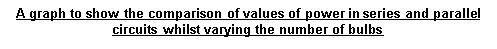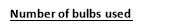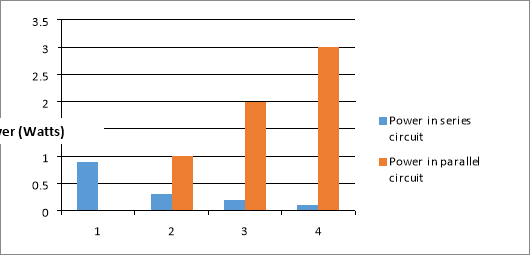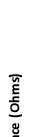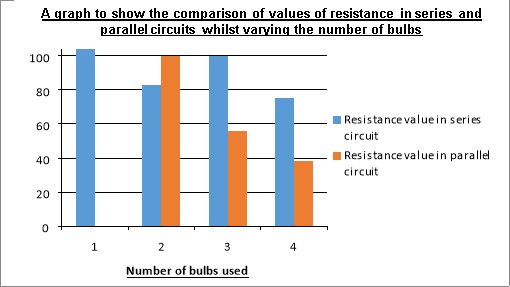The findings show that for the series circuits show that as the number of bulbs increases the value of power and resistance both decrease. However, when there were 3 and 4 bulbs within the circuit the value of resistance remained the same within the circuit which doesn’t fit in with the general trend, therefore figure 3 or 4 may be an anomaly.

The calculations show for the parallel circuits show that as the number of bulbs increase the value of electrical power increases, whilst the value of resistance decreases.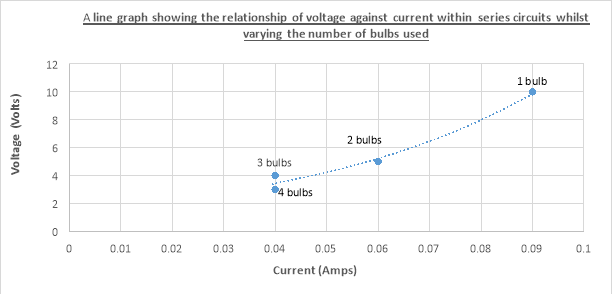This graph shows that ohms law has been obeyed as the current flowing through is quiet proportional to the potential difference across.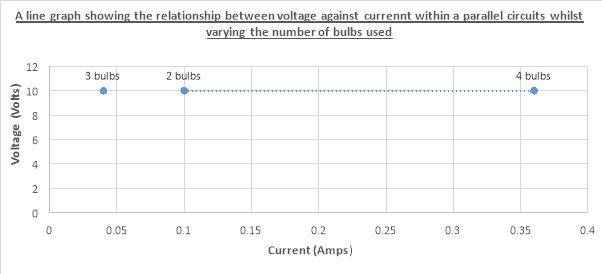Evaluation:

There may have been one anomaly that was recorded within the series circuits between the 3 and 4 bulbs experiment as the current should have decreased but stayed the same. However this could have been because a needle ammeter was used, therefore is subject to one’s opinion thus not entirely accurate, in order to improve this a digital ammeter could have been used to improve reliability.

Also the ammeter scale couldn’t be accurately read as there was no gradation between the 1 and 2 values.

In order to improve the method more number of bulbs could have been used to show more of an overall trend which would improve validity and reliability of conclusion. In order to improve the reliability and validity of the method a repeat should be conducted of the experiment to achieve average results. Overall, the results match theory thus portraying the results achieved are reliable.

View all

## DMCA / Removal Request

If you are the original writer of this essay and no longer wish to have your work published on UKEssays.com then please:

Related Services

Prices from

S\$203

Approximate costs for:

• 1000 words
• 7 day deliveryHumanity University

Dedicated to your worth and value as a human being!

Related Lectures